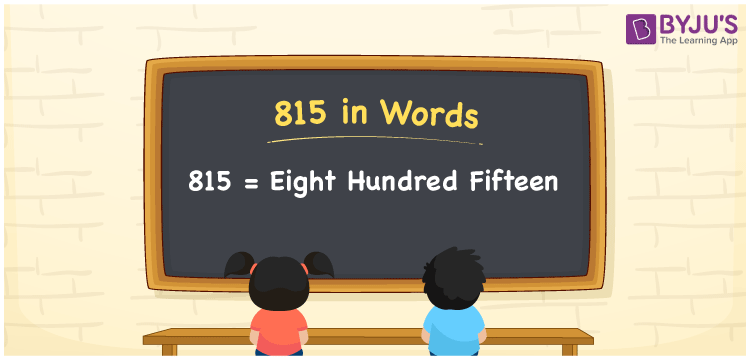# 815 in words

815 in words is written as Eight Hundred and Fifteen. 815 represents the count or value. The article on Counting Numbers can give you an idea about count or counting. The number 815 is a 3 digit number that is used in expressions related to money, days, distance, length, weight and so on. Let us consider an example for 815. “I have a collection of Eight Hundred and Fifteen books”.

 815 in words Eight Hundred and Fifteen Eight Hundred and Fifteen in Numbers 815

## 815 in English Words## How to Write 815 in Words?

We can convert 815 to words using a place value chart. The number 815 has 3 digits, so let’s make a chart that shows the place value up to 3 digits.

 Hundreds Tens Ones 8 1 5

Thus, we can write the expanded form as:

8 × Hundred + 1 × Ten + 5 × One

= 8 × 100 + 1 × 10 + 5 × 1

= 815

= Eight Hundred and Fifteen.

815 is the natural number that is succeeded by 814 and preceded by 816.

815 in words – Eight Hundred and Fifteen.

Is 815 an odd number? – Yes.

Is 815 an even number? – No.

Is 815 a perfect square number? – No.

Is 815 a perfect cube number? – No.

Is 815 a prime number? – No.

Is 815 a composite number? – Yes.

## Solved Example

1. Write the number 815 in expanded form

Solution: 8 × 100 + 1 × 10 + 5 × 1

We can write 815 = 800 + 10 + 5

= 8 × 100 + 1 × 10 + 5 × 1.

## Frequently Asked Questions on 815 in words

Q1

### How to write the number 815 in words?

815 in words is written as Eight Hundred and Fifteen.
Q2

### Is 815 divisible by 5?

Yes. 815 is divisible by 5.
Q3

### Is 815 a prime number?

No. 815 is not a prime number.